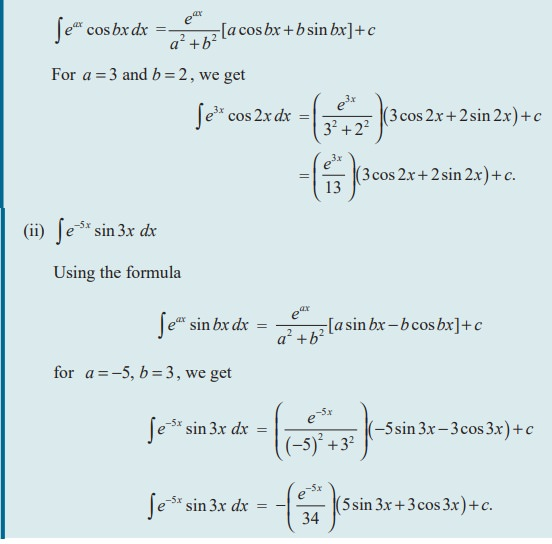Home | | Maths 11th std | Integrals of the form e pow(ax) cos bx and e pow(ax) sin bx

# Integrals of the form e pow(ax) cos bx and e pow(ax) sin bx

The following examples illustrate that there are some integrals whose integration continues forever.

## (i) Ōł½eaxaxsinbx dx (ii) Ōł½eaxcosbx dx

The following examples illustrate that there are some integrals whose integration continues forever. Whenever we integrate function of the form e axcos bx or eax sinbx, we have to apply the Integration by Parts rule twice to get the similar integral on both sides to solve.

Result 11.1### Caution

In applying integration by parts to specific integrals, the pair of choice for u and dv once initially assumed should be maintained for the successive integrals on the right hand side. (See the above two examples). The pair of choice should not be interchanged.

Examples 11.36

Evaluate the following integrals

(i) Ōł½e 3x cos 2x dx

(ii) Ōł½e ŌłÆ5x sin 3x dx

(i) Ōł½e 3x cos 2x dx

Using the formula### EXERCISE 11.8

Integrate the following with respect to x

(1) (i) eax cos bx (ii) e2 x sin x (iii) eŌłÆ x cos 2x

(2)  (i) eŌłÆ3x sin 2x (ii) eŌłÆ4xsin 2x (iii) eŌłÆ3x cos x

### Result 11.2

Ōł½ex [ f (x) + f ŌĆ▓(x)]dx = ex f (x) + c

### Proof

Let I = Ōł½ex [ f (x) + f ŌĆ▓(x)]dx

= Ōł½ e x f (x)dx + Ōł½ex f ŌĆ▓(x)dx

Take u = f ( x); du =  fŌĆÖ ( x) dx, in the first integral

dv = ex ;v = ex ,

That is, I = ex f (x) ŌłÆ Ōł½ e x f ŌĆ▓( x)dx + Ōł½ex f ŌĆ▓(x)dx + c

Therefore, I = e x f ( x ) + c.

### EXERCISE 11.9

Integrate the following with respect to x:Tags : Equation, Solved Example Problems | Mathematics , 11th Mathematics : UNIT 11 : Integral Calculus
Study Material, Lecturing Notes, Assignment, Reference, Wiki description explanation, brief detail
11th Mathematics : UNIT 11 : Integral Calculus : Integrals of the form e pow(ax) cos bx and e pow(ax) sin bx | Equation, Solved Example Problems | Mathematics